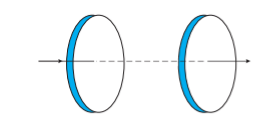Home/Class 12/Physics/

## QuestionPhysicsClass 12

Figure shows a capacitor made of two circular plates each of radius $$12\text{ cm}$$, and separated by $$5.0\;\text{cm}.$$ The capacitor is being charged by an external source (not shown in the figure). The charging current is constant and equal to $$0.15\;A$$. Is Kirchhoff’s first rule $$($$junction rule$$)$$ valid at each plate of the capacitor? Explain.Yes, Kirchhoff’s first junction rule is valid at each plate of the capacitor provided that we take the sum of conduction and displacement for current.